# ALSO - At what (x,y) position is the electric field (21,600ı^-28,800ȷ^) N/C? Enter the x and...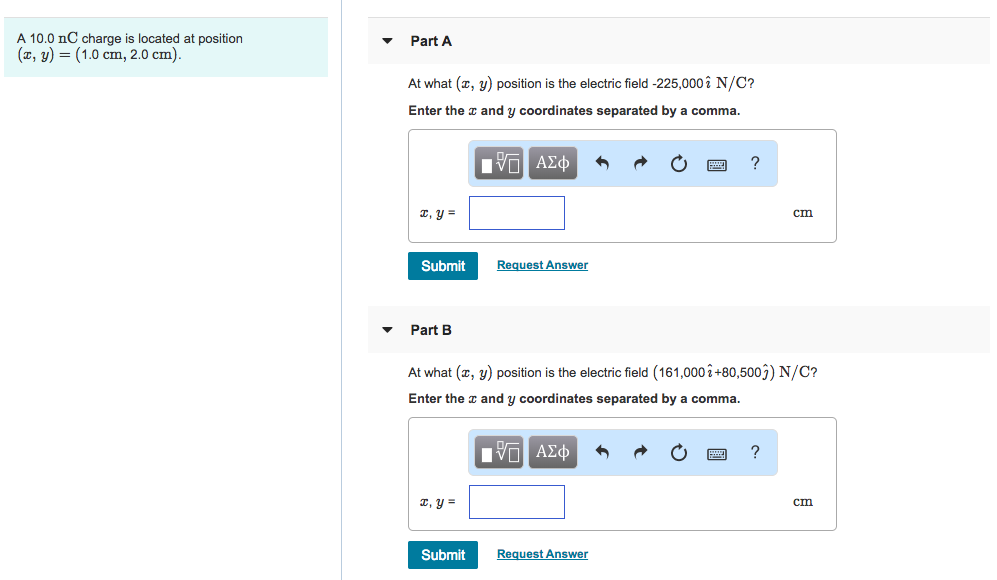ALSO - At what (x,y) position is the electric field (21,600ı^-28,800ȷ^) N/C?

Enter the x and y coordinates separated by a comma.

A 10.0 nC charge is located at position (z, y) = (1.0 cm, 2.0 cm). ▼ Part A At what (x, y) position is the electric field -225,000i N/C? Enter the and y coordinates separated by a comma. cm Submit Request Answer ▼ Part B At what (x, y) position is the electric field (161,000 i+80,500j) N/C? Enter the and y coordinates separated by a comma. cm Submit Request Answer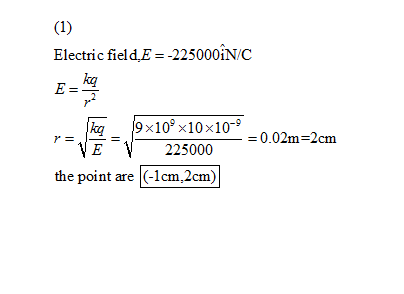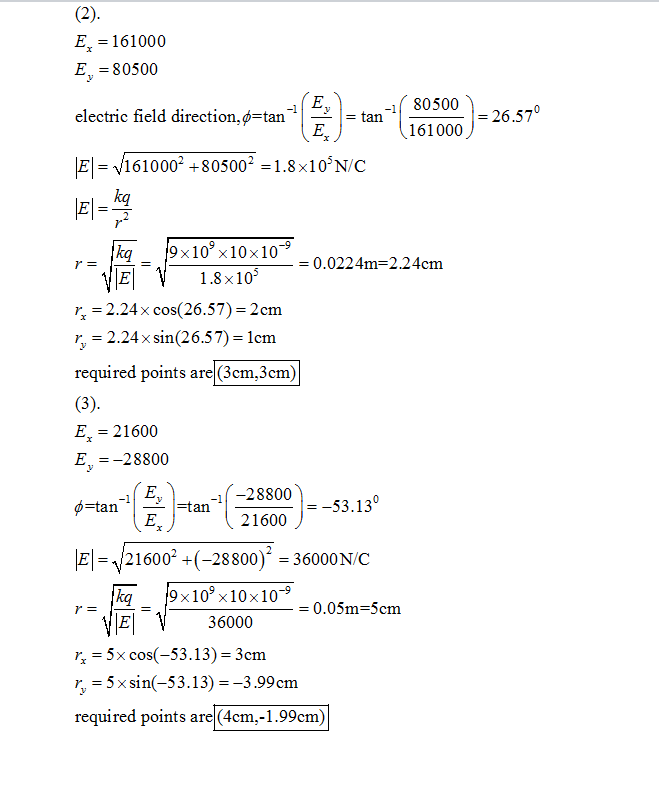Please rate my answer, good luck...

##### Add Answer to: ALSO - At what (x,y) position is the electric field (21,600ı^-28,800ȷ^) N/C? Enter the x and...
Similar Homework Help Questions
• ### Homework-1 Problem 25.63 8 of 8 Review Part A A-10.0 nC charge is located at position...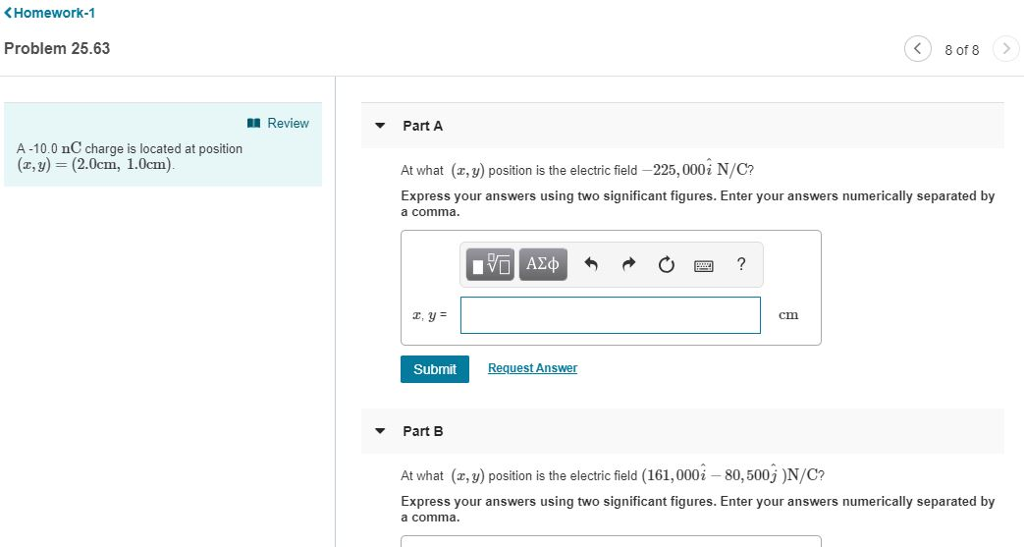Homework-1 Problem 25.63 8 of 8 Review Part A A-10.0 nC charge is located at position (,) (2.0cm, 1.0cm) At what (z,y) position is the electric field-225,000İN/C? Express your answers using two significant figures. Enter your answers numerically separated by a comma cm Submit uest Ans Part B At what (z,y) position is the electric field (161,0002-80,500)N/C? Express your answers using two significant figures. Enter your answers numerically separated by a comma. Homework-1 Problem 25.63 8 of 8 0四 I...

• ### Part C What is the electric field at point 3 in the figure? Give your answer...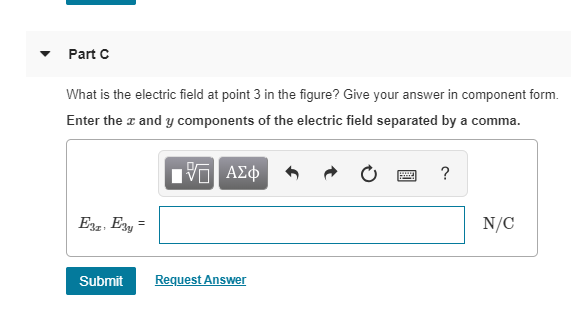Part C What is the electric field at point 3 in the figure? Give your answer in component form. Enter the z and y components of the electric field separated by a comma. N/C Submit RequestAnswer Review Part A What is the electric field at point 1 in (Figure 1)? Give your answer in component form Assume that a 2.9 cm and b 3.6 cm. Enter the r and y components of the electric field separated by a comma N/C...

• ### Review Part A What is the electric field at point 1 in (Figure 1)? Give your...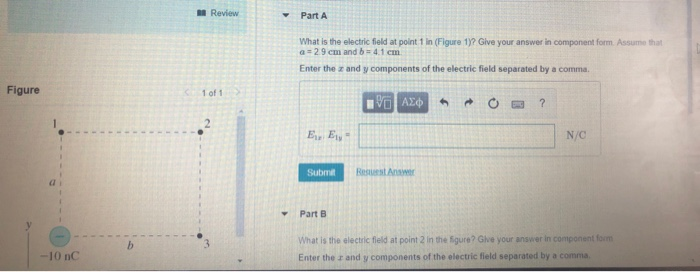Review Part A What is the electric field at point 1 in (Figure 1)? Give your answer in component form Assume that 29cm and b 4 1 cm Enter the z and y components of the electric field separated by a comma Figure 1 of 1 N/C Resuest Ans a i Part B What is the electric fleld at point 2 in the 5gure? Give your answer in component fom Enter the r and y components of the electric field...

• ### Finding position given electric field and charge

A - 10.0 nC charge is located at position ( x, y ) = ( 2.0cm;1.0 cm ) . At what ( x, y) position(s) is the electric field 161000i - 80500j N/C? 28800i + 21600jN/C?

• ### Problem 22.63 11 of 11 Review Part A what is the electric field at point 1...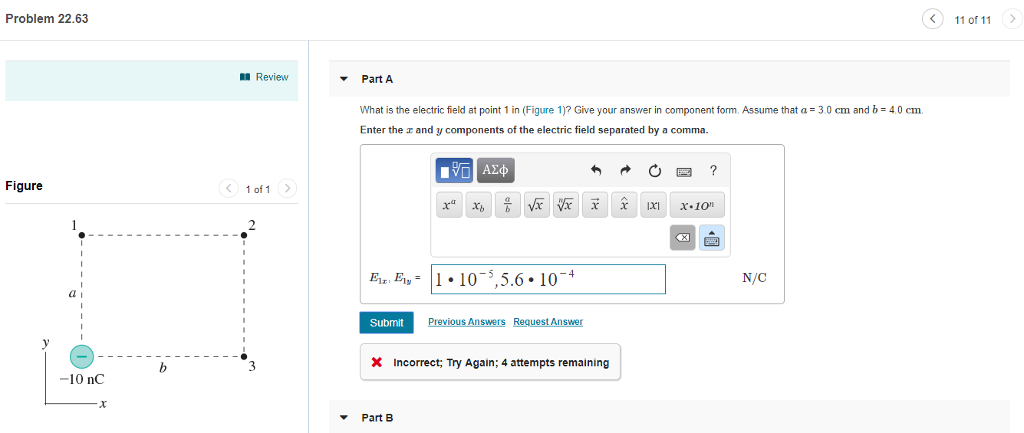Problem 22.63 11 of 11 Review Part A what is the electric field at point 1 in (Figure 1)? Give your answer in component form Assume that a = 3 0 cm and b = 4.0 cm Enter the and y components of the electric field separated by a comma. Figure < 1of1 N/C Submit X Incorrect; Try Again; 4 attempts remaining -10 nC Part B Problem 22.63 11 of 11 Review Part B What is the electric field at...

• ### Item 14 Review PartA What is the electric field at point 1 in (Figure 1)? Give...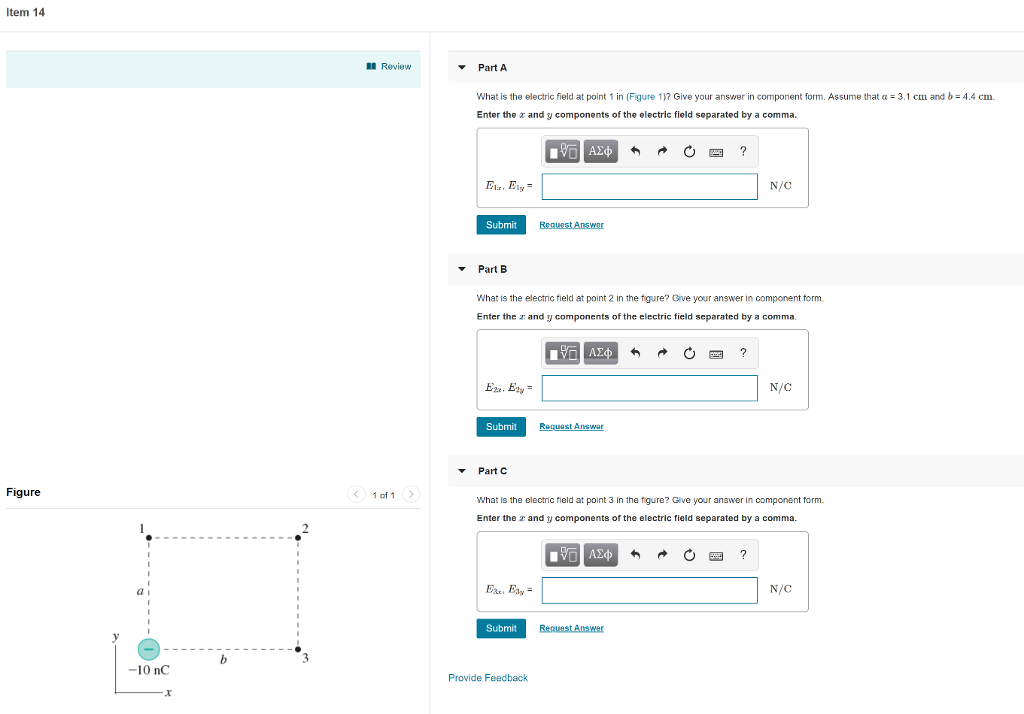Item 14 Review PartA What is the electric field at point 1 in (Figure 1)? Give your answer in component form. Assume that α = 3.1 cm and b= 4.4 cm. Enter the x and y components of the electric field separated by a comma. Etr, Ely N/C Submit Part B What is the electric field at point 2 in the figure? Give your answer in component form Enter the and y components of the electric field separated by a...

• ### Item 14 Review PartA What is the electric field at point 1 in (Figure 1)? Give...Item 14 Review PartA What is the electric field at point 1 in (Figure 1)? Give your answer in component form. Assume that α = 3.1 cm and b= 4.4 cm. Enter the x and y components of the electric field separated by a comma. Etr, Ely N/C Submit Part B What is the electric field at point 2 in the figure? Give your answer in component form Enter the and y components of the electric field separated by a...

• ### Review Constants Part A A +15 nC charge is located at (x, y) (0 cm, 9.0...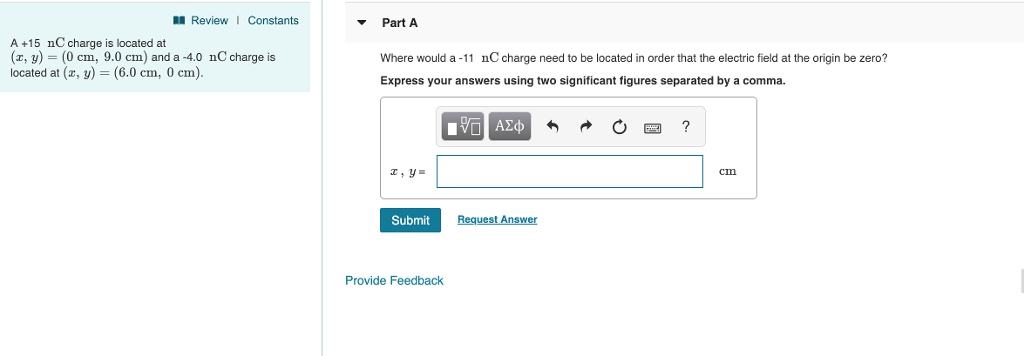Review Constants Part A A +15 nC charge is located at (x, y) (0 cm, 9.0 cm) and a -4.0 nC charge is located at (z, y) 6.0 cm, 0 cm). Where would a-11 nC charge need to be located in order that the electric field at the origin be zero? Express your answers using two significant figures separated by a comma. cm Submit Request Arn sWe Provide Feedback

• ### What is the z-component of the electric field at the position (z. У)-(0 cm, 5 4...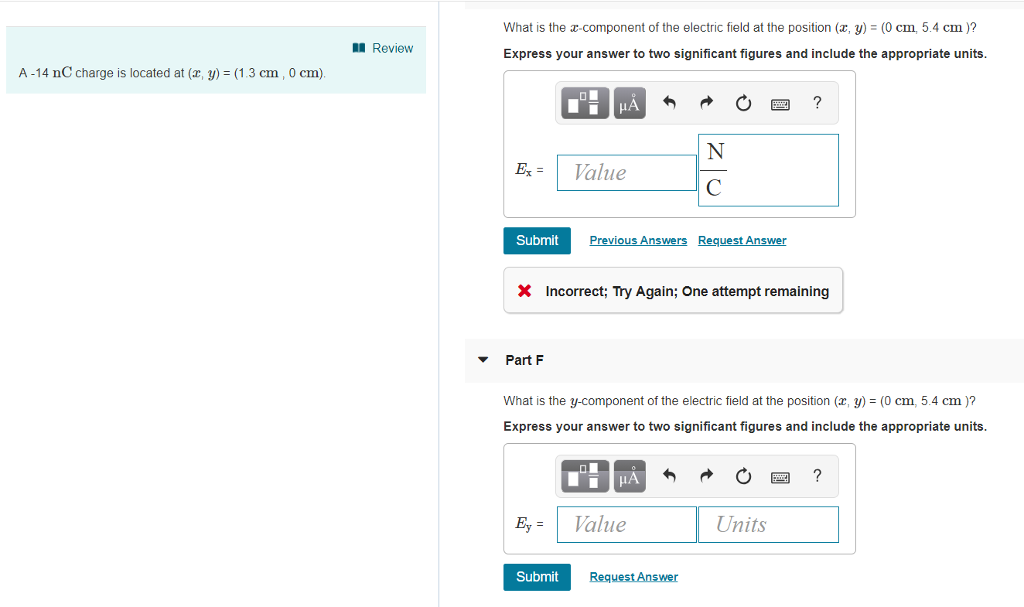What is the z-component of the electric field at the position (z. У)-(0 cm, 5 4 cm )? I Review Express your answer to two significant figures and include the appropriate units A-14 nC charge is located at (2, y) = (1.3 cm , 0 cm) E-1 Value Submit ns X Incorrect; Try Again; One attempt remaining PartF what is the y-component of the electric field at the position (z. y) : (0 cm, 5 4 cm )? Express your...

• ### Help with parts a-c thanks! A-14 nC charge is located at the ori orngin What is...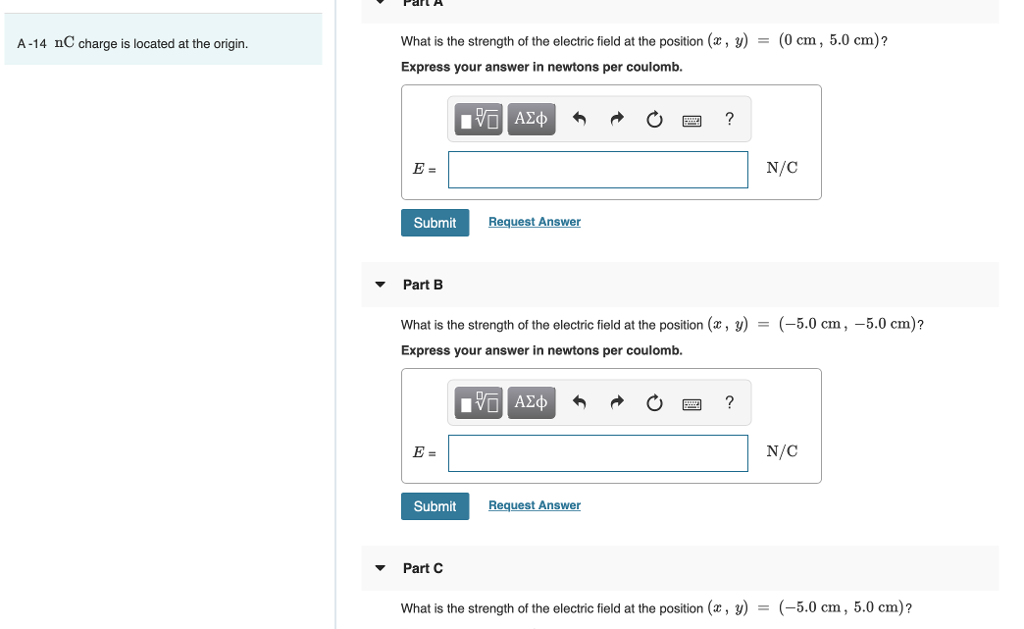Help with parts a-c thanks! A-14 nC charge is located at the ori orngin What is the strength of the electric field at the position (x, y)- (0 cm, 5.0 cm)? Express your answer in newtons per coulomb. N/C Submit Request Answer Part B What is the strength of the electric field at the position (x, y)- Express your answer in newtons per coulomb. (-5.0 cm, -5.0 cm)? N/C Submit Request Answer Part C What is the strength of the...

Free Homework App